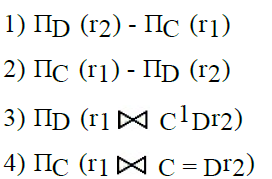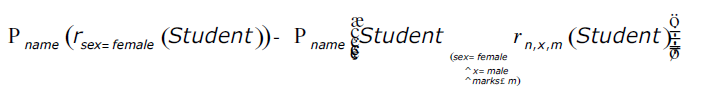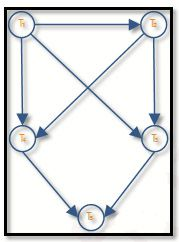91. Given the following input (4322, 1334, 1471, 9679, 1989, 6171, 6173, 4199) and the hash function x mod 10, which of the following statements are true?1. 9679, 1989, 4199 hash to the same value2. 1471, 6171 hash to the same value3. All elements hash to the same value4. Each element hashes to a different value a. 1 only b. 2 only c. 1 and 2 only d. 3 or 4

 92. Let R1 (A, B, C) and R2 (D, E) be two relation schema, where the primary keys are shown underlined, and let C be a foreign key in R1 referring to R2. Suppose there is no violation of the above referential integrity constraint in the corresponding relation instances r1 and r2. Which one of the following relational algebra expressions would necessarily produce an empty relation ?a. 1 b. 2 c. 3 d. 4

 93. Consider the relation Student (name, sex, marks), where the primary key is name, pertaining to students in a class that has at least one boy and one girl. What does the following relational algebra expression produce? (Note: r is the rename operator). The condition in join is "(sex = female ^ x = male ^ marks ≤ m)"a. names of girl students with the highest marks b. names of girl students with more marks than some boy student c. names of girl students with marks not less than some boy students4) d. names of girl students with more marks than all the boy students

 94. How many serial schedules are possible using n-transactions? a. (n-1)! b. n! c. n(n-1)! d. n(n+1)! / 2

 95. Which of the following is wrong? The goal of concurrent execution is a. Improved Throughput b. Reduced Probability of Deadlock c. Improved resource Utilization d. Reduced waiting time

 96. Consider the following ordering of transactions: T1 : R(X); T2 : R(X); T1 : w(x); T1 : r(y); T2 : w(x); T2 : Commit; T1: w(y) ; T1: Commit Which of the following is true? a. It is a strict schedule b. Cascade less schedule c. Irrecoverable d. Recoverable

 97. In strict two-phase locking protocol a. All exclusive mode lock taken by transaction be held until transaction commits b. All exclusive mode locks taken by transaction can be released before transaction commits c. All locks can be released before transaction commits d. None of these
 98. Is the corresponding schedule is conflict serializable schedule?a. Yes b. No c. Can't say d. None of these